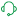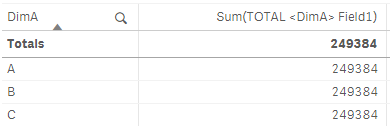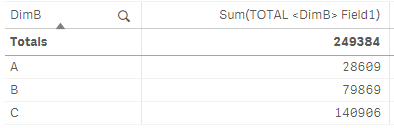# Knowledge

Search or browse our knowledge base to find answers to your questions ranging from account questions to troubleshooting error messages. The content is curated and updated by our global Support team

Announcements
Support Case Portal has moved to Qlik Community! Read the FAQs to start exploring Support resources.

# Sum(TOTAL <Dim> Field) Does Not Return The Expected Partial Sum Per Dimensional ValueSupport

## Sum(TOTAL <Dim> Field) Does Not Return The Expected Partial Sum Per Dimensional Value

Aggregated total is not the expected partial sum per dimensional value. This issue can be seen with any aggregation function that allows for total field qualifier, i.e. Sum(TOTAL <fld {,fld}> expr).

In this scenario, the calculated dimension is used to reformat an existing field in the data model. The dimension is given the same label as the original field name.

For example the dimension DimA is reformatted to =Upper(DimA) and with the label DimA. This synthetic dimension is used as field qualifier reference in an aggregation like Sum(TOTAL <DimA> Field1)
The aggregated result per dimensional value is unexpectedly a sum total, e.g. the same as the column total of the measure.The expected result is a partial sum per dimensional value.#### Cause:

Dimensions get a default labels with is the same as the dimension definition. Calculated dimensions, therefore, get a label that is the actual expression.This means that field qualifier in measure and dimension label are different. The aggregated result can not be divided over the dimensional values, as the referred field label does not exist in the visualization.The calculated dimension can be given a suitable label manually. If the label is the same as an existing field in the data model, the Qlik Sense engine can not determine which field to pick, so the aggregated result becomes the expression total.This means that the result for each dimensional value is the same as the measure being aggregated without any dimensions. This result can be compared with aggregating the measure in a text object or visualization title, where the aggregation does not have a dimensional context.

Sum(TOTAL <DimA> Field1) = 249384

### Resolution:

Total field qualifier must refer unique field name or label, and the same unique label must be used in the chart.

The best practice recommendation is to apply preferred field formats during the data load. This minimizes the need to alter the format in multiple locations in-app sheets and master items. It also means usage of fewer calculated dimensions in apps, which optimizes calculation effort during user consumption.

Alternatively, the calculated dimension must be given a unique label, which does not match an existing field in the data model. For example, the calculated dimension has been given the label DimB, which is not the name of an existing field in the data model. The same label is then used as field qualifier in the total aggregation.Labels (1)
• ### App Development

Version history
Revision #:
2 of 2
Last update:
‎2020-10-15 03:56 AM
Updated by:Contributors